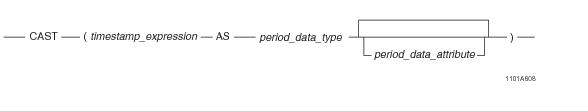# 15.00 - TIMESTAMP-to-Period Conversion - Teradata Database

## Teradata Database SQL Functions, Operators, Expressions, and Predicates

Product
Release Number
15.00
Content Type
Programming Reference
Publication ID
B035-1145-015K
Language
English (United States)

## TIMESTAMP-to-Period Conversion

### Purpose

Converts a TIMESTAMP value as PERIOD(DATE), PERIOD(TIME[(n)][WITH TIME ZONE]), or PERIOD(TIMESTAMP[(n)][WITH TIME ZONE]).

### CAST Syntaxwhere:

 Syntax element … Specifies … timestamp_expression the TIMESTAMP data expression to be converted. period_data_type the target Period type to which timestamp_expression is to be converted. period_data_attribute one of the following optional data attributes: FORMAT NAMED TITLE

### ANSI Compliance

This is ANSI SQL:2011 compliant.

As an extension to ANSI, CAST permits the use of data attribute phrases.

### Usage Notes

A TIMESTAMP(n) [WITH TIME ZONE] value can be cast as PERIOD(DATE), PERIOD(TIME[(n)] [WITH TIME ZONE]), or PERIOD(TIMESTAMP[(n)] [WITH TIME ZONE]) using the CAST function.

If the target type is PERIOD(TIME[(n)] [WITH TIME ZONE]) or PERIOD(TIMESTAMP[(n)] [WITH TIME ZONE]):

• If the target precision is higher than the source precision, trailing zeros are added in the result bounds to adjust the precision.
• If the target precision is lower than the source precision, an error is reported.
• If the target type is PERIOD(DATE), the result beginning bound is the date portion of the source beginning bound adjusted to the current session time zone.

If the target type is PERIOD(TIME[(n)]), the result beginning bound is the time portion of the source value (in UTC).

If the target type is PERIOD(TIME[(n)] WITH TIME ZONE), the result beginning bound is formed from the time portion of the source value (in UTC) and, if the source type is WITH TIME ZONE, the source time zone displacement and, if not, the current session time zone displacement.

If the target type is PERIOD(TIMESTAMP[(n)]), the result beginning bound is the timestamp portion of the source value (in UTC).

If the target type is PERIOD(TIMESTAMP[(n)] WITH TIME ZONE), the result beginning bound is formed from the timestamp portion of the source value (in UTC) and, if the source type is WITH TIME ZONE, the source time zone displacement and, if not, the current session time zone displacement.

If the TIMESTAMP source value contains leap seconds, the seconds portion gets adjusted to 59.999999 with the precision truncated to the target precision.

The result ending element is set to the result beginning bound plus one granule of the target type. If the result ending bound exceeds the maximum allowed DATE or TIMESTAMP value for a target type of PERIOD(DATE) or PERIOD(TIMESTAMP[(n)]), respectively, or the ending bound has a lower value than the result beginning bound in their UTC forms for a target type of PERIOD(TIME[(n)]), an error is reported.

Note: If the target type is WITH TIME ZONE, the result beginning and ending bounds have the same time zones.

Also, note that the result has the same value for the beginning bound and last value.

### Example

In the following example, a TIMESTAMP(6) literal is cast as PERIOD(DATE). The result beginning element is set to the date portion of the source value. The result ending element is set to result beginning bound plus INTERVAL '1' DAY.

`   SELECT CAST(TIMESTAMP '2005-02-03 12:12:12.340000' AS PERIOD(DATE));`

The following is returned:

`   ('2005-02-03', '2005-02-04')`

### Related Topics

For details on data types and data attributes, see SQL Data Types and Literals.